×

Search anything:

# Binary Search Tree with Parent Pointer

#### Data Structures Problems on Binary TreeIn this article, we have covered Binary Search Tree with Parent Pointer which is a modification of the standard Data Structure, Binary Tree.

1. Introduction
2. Binary Search Tree data structure with Parent Pointer
3. Implementation Example
4. Time & Space Complexity
5. Applications

# Introduction

A Binary Search Tree (BST) is a data structure where we store values in a tree form. Each node has a value and two child nodes associated with it. The left child node has a value smaller than the parent node, and the child node in the right contains a value greater than the parent node.

At the top of the tree, we have the root node. All nodes smaller than the root node's value lie towards the left of the root node. These values make the left subtree. All nodes greater than the root node lie towards the right of the root node. They make the right subtree. The following image shows an example of a Binary Search Tree.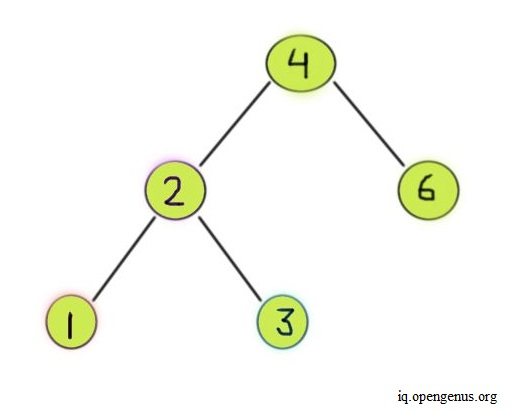If the difference between the height of the left and right subtrees of every node is not more than one, then it's called a Balanced Binary Search Tree (as shown in the image above). Otherwise, we consider the BST as an unbalanced BST.

The following image shows an example of an unbalanced BST.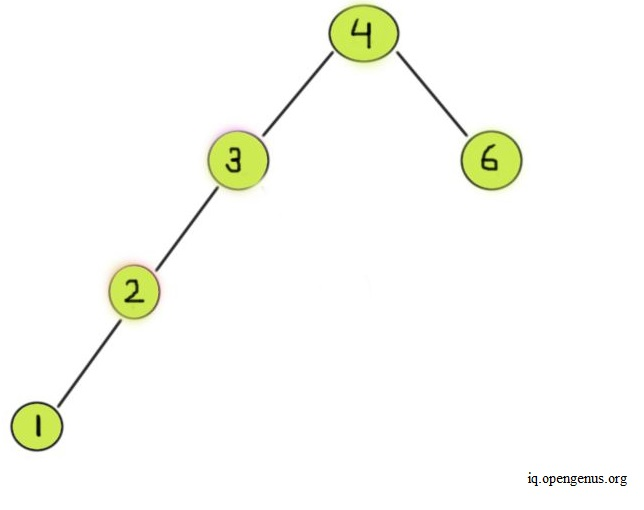We create a BST with a parent pointer for each node. This way, we can access the parent and child nodes conveniently.

# Binary Search Tree data structure with Parent Pointer

Each instance of a BST node contains a value, along with a value each for its left and right child nodes. We also save the value for the node's parent. To implement a BST with parent pointer, we create the following -

A class for the BST node
We create a class for a BST node as shown in the code below.

``````# a class to create a node structure
class new_node:
def __init__(self, item):
self.key = item
self.left = self.right = None
self.parent = None
``````

For the class attributes, create one each for the value of the node itself, the left child node, the right child node, and the node's parent. Initially let the left, right, and the parent nodes be of the `None` type.

A function to insert a node
To insert a node into the BST, we create a utility function. As shown in the code below, we set two parameters for the function, a node, and a key.

``````def insert_node(node, key):

# If the tree is empty, we return a new node
if node == None:
return new_node(key)

# Otherwise, perform recursion down the tree
if key < node.key:
node.left = insert_node(node.left, key)

# Set current root node
# as parent of left child node
node.left.parent = node

elif key > node.key:
node.right = insert_node(node.right, key)

# Set current root node
# as parent of right child node
node.right.parent = node

# return the node pointer
return node
``````

The node parameter takes care of the root of the BST and the subsequent nodes inside it, and we use the key for the value to be inserted and for comparing it with the nodes (if any) inside the BST. We first check if the tree is empty and if it is, we convert the key into an instance of the node class and return it. This node becomes the root of our tree. In case the tree is not empty, we check the key value with the node's value and it's less, then we recurse to return this key as a node object and set it as the root node's left child node. We also set the parent of the left child node. Likewise, we do the same for the right child node. At the end of the function, we return the current node whose left and right child nodes we just set.

A function to find the smallest valued node
We also create a utility function to find the smallest valued node.

``````def smallestnode(node):

# loop down to find the leftmost leaf as
# leftmost leaf value is the smallest node

while(node.left is not None):
node = node.left

return node
``````

Depending on the node we pass into this function, we continue to loop down its left most value, and stop if we reach a value in the tree that is of the `None` type. In the end, we return the last left most node value.

A function to delete a node in the BST
Whenever we want to delete a node, we face three cases: the node to be removed will have no child nodes, one child node, or two child nodes. The code below shows how we delete a node in each of these cases.

``````def delete_node(root, key):

# Base Case
if root is None:
return root

# If the key to be deleted
# is less than the root key
# recur down the left subtree
if key < root.key:
root.left = delete_node(root.left, key)
if root.left:
root.left.parent = root

# If the kye to be deleted
# is greater than the root's key
# recur down the right subtree
elif(key > root.key):
root.right = delete_node(root.right, key)
if root.right:
root.right.parent = root

# If key is equal to root's key then
# this is the node we have to delete

else:

# Node with only one child or no child
if root.left is None:
temp = root.right
return temp

elif root.right is None:
temp = root.left
return temp

# Node with two children:
# Get the inorder successor, which is the
# smallest valued node in the right subtree
temp = smallestnode(root.right)

# Replace the key of the node to be deleted
# with its inorder successor's key
root.key = temp.key

# Remove the inorder successor after
# switching it with the node to be deleted
root.right = delete_node(root.right, temp.key)

return root

``````

In case the node has no child nodes, we can simply remove the node without having any impact on the rest of the BST. In case the node to be removed has one child, we store its child node in a temporary variable, set the node to be deleted as `None`, and return the value of the temporary variable. In case the node to be deleted has no children, the temporary variable will have `None` as its value. This node is returned back to the previous stack, where it's set in place of the node to be deleted. So, we replace the node to be deleted with its child node. After replacing, we check if this returned node is `None` and if it is not, we set its parent equal to the root node in the current recursion stack. This way we can delete a node with one child.

In case the node has two child nodes, we decide with which child node we're going to replace it. We can choose either its in-order successor or its in-order predecessor. The in-order successor of a particular node is the node that comes immediately after this particular node whenever we traverse the BST in ascending order, the smallest valued node to the biggest valued node. The in-order predecessor of a node is the node that comes immediately before it during the in-order traversal of the BST. In the image below, the in-order predecessor of node 2 is 1 (shown in red) and the in-order successor is 3 (in blue).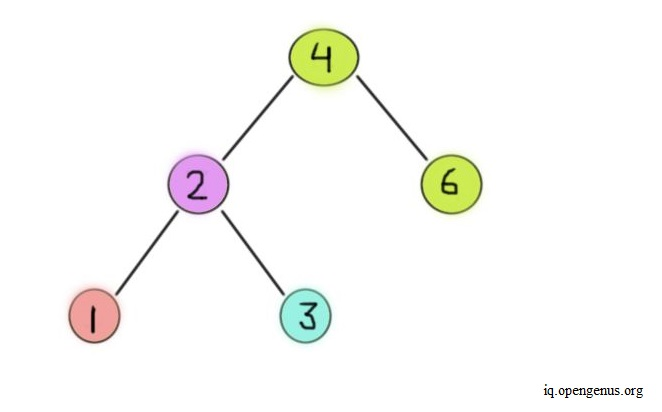In this case, we have used the in-order successor to replace the node to be deleted. We again use a temporary variable to store the in-order successor of the node to be removed. To find the in-order successor we use the utility function we created in the previous step. After this, we replace the key of the node to be removed with the key of its in-order successor. Then, we remove the in-order successor of the node and place it with a value of `None`.

A function to traverse the BST in order
We then create a function to perform in-order traversal of the BST. This helps us to print the BST and its nodes in ascending order.

``````
def inorder_trav(root):

# check if root is None
if root != None:

# find the smallest node
inorder_trav(root.left)

# print the node
print("Node :", root.key, ", ", end = "")

# print the parent node
if root.parent == None:
print("Parent node: NULL")
else:
print("Parent node: ", root.parent.key)

# check the right child node
inorder_trav(root.right)

``````

We pass the root node of the BST and check if it's `None`. In case it isn't, we recurse till we've reached the latest leftmost node in the entire tree, as this node will have the smallest value. We print this left node's key and check if its parent node is `None`. We print the parent node's key if it exists, otherwise, we print `NULL`. Then, we recurse again to check this left node's right node. If it's not `None`, we do the same as we did for the leftmost node. Otherwise, if it's `None`, the recursion for this node ends and we go back to this left node's parent node and repeat the same process as we did for the leftmost node. This continues till we've traversed the entire BST. The image below shows the order in which the in-order traversal occurs for each node in the BST.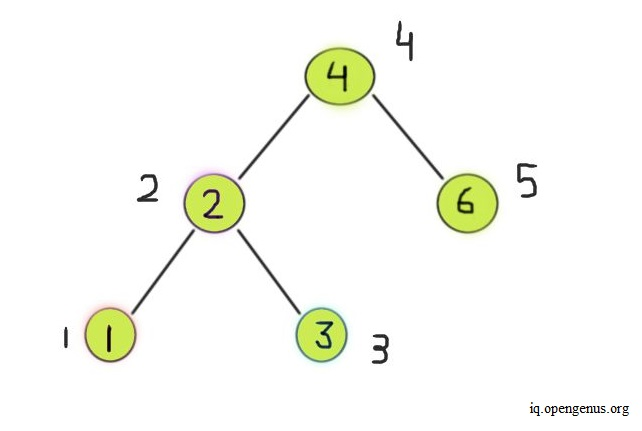# Implementation Example

After combining the classes and functions discussed above, we can use the following program to create a BST with parent pointers.

``````
# a class to create a node structure
class new_node:
def __init__(self, item):
self.key = item
self.left = self.right = None
self.parent = None

# A  function to insert a new node given a key, in BST

def insert_node(node, key):

# If the tree is empty, we return a new node
if node == None:
return new_node(key)

# Otherwise, perform recursion down the tree
if key < node.key:
node.left = insert_node(node.left, key)

# Set current root node
# as parent of left child node
node.left.parent = node

elif key > node.key:
node.right = insert_node(node.right, key)

# Set current root node
# as parent of right child node
node.right.parent = node

# return the node pointer
return node

def smallestnode(node):

# loop down to find the leftmost leaf as
# leftmost leaf value is the smallest node

while(node.left is not None):
node = node.left

return node

# a function to delete a node in BST
# given a key and BST's root

def delete_node(root, key):

# Base Case
if root is None:
return root

# If the key to be deleted
# is less than the root key
# recur down the left subtree
if key < root.key:
root.left = delete_node(root.left, key)
if root.left:
root.left.parent = root

# If the kye to be deleted
# is greater than the root's key
# recur down the right subtree
elif(key > root.key):
root.right = delete_node(root.right, key)
if root.right:
root.right.parent = root

# If key is equal to root's key then
# this is the node we have to delete

else:

# Node with only one child or no child
if root.left is None:
temp = root.right
return temp

elif root.right is None:
temp = root.left
return temp

# Node with two children:
# Get the inorder successor, which is the
# smallest valued node in the right subtree
temp = smallestnode(root.right)

# Replace the key of the node to be deleted
# with its inorder successor's key
root.key = temp.key

# Remove the inorder successor after
# switching it with the node to be deleted
root.right = delete_node(root.right, temp.key)

return root

# A function to do inorder traversal of BST

def inorder_trav(root):

# check if root is None
if root != None:

# find the smallest node
inorder_trav(root.left)

# print the node
print("Node :", root.key, ", ", end = "")

# print the parent node
if root.parent == None:
print("Parent node: NULL")
else:
print("Parent node: ", root.parent.key)

# check the right child node
inorder_trav(root.right)

# An example BST to test above program
if __name__ == '__main__':

# We create the following BST
#         40
#       /   \
#      20   60
#     / \   / \
#   10 30  50 70

keys = [40,20,10,30,60,50,70]

root = None
for key in keys:
root = insert_node(root, key)

# delete node 20
root = delete_node(root,20)
# print inorder traversal of the BST
inorder_trav(root)

``````

In the example BST that we create above, we have a root node of the `None` type in the beginning. Later, we pass the root node with the rest of the keys we want to insert, one by one. Since 40 is the first key we pass into the `insert_node` function, 40 gets set as the root node of the BST. Then using 40 as root, we insert the rest of the keys as nodes into our BST. At this stage, our BST looks like the image shown below.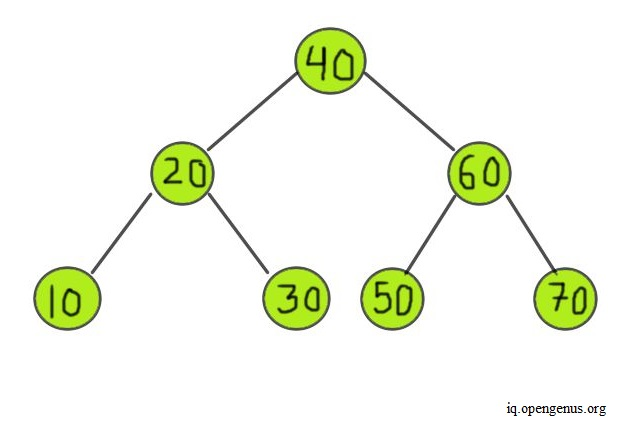Then, we call the `delete_node` function to delete node 20. Since 20 is a node that has two children, we choose 20's in-order successor node, node 30. We replace node 20's key with 30 and then set node 20's right child node as `None`. This way, we delete node 20 and replace it with its in-order successor. At the end of the entire program, our final BST has the following structure -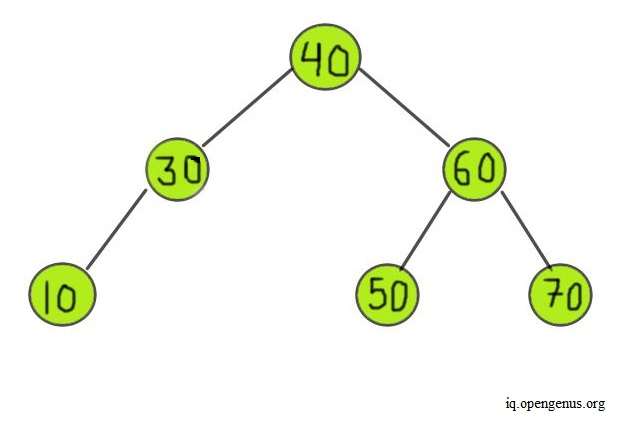When we run the above program, we get the following output-

``````
Node : 10 , Parent node:  30
Node : 30 , Parent node:  40
Node : 40 , Parent node: NULL
Node : 50 , Parent node:  60
Node : 60 , Parent node:  40
Node : 70 , Parent node:  60

``````

# Time & Space Complexity

• Time complexity: The overall time complexity of implementing this data structure is O(n) in the worst case as sometimes, the BST we create can become unbalanced. So in such a case, the BST structure is much like a linked list, and we have to traverse each node for insert, search, and deletion operations. In the best case, we'd have a runtime of O(log(n)) for all operations, where the BST is balanced and the height of every subtree is uniform and doesn't differ by more than 1.

• Space complexity: In both the best and worst cases, the space required to create this structure is O(n), as the size of the tree depends on the number of nodes inside it.

# Applications

Binary Search Trees are useful for a variety of applications-

• Indexing and Searching: We can use a BST to create an index for a database, which we can then use to access records from the said database quickly.

• Sorting: BSTs are also helpful for sorting large amounts of data. We can insert all data points in a BST and then perform in-order traversal to get the data in sorted order.

• Maintaining Priority Queues: using the key of the nodes as priorities, we can use a BST structure to maintain priority queues.

With this article at OpenGenus, you must have the complete idea of Binary Search Tree with Parent Pointer.